# Reduce LED Noise and Distortion for Accurate Pulse Oximetry

By Bonnie Baker

Contributed By Digi-Key's North American Editors

Pulse oximetry is a noninvasive way to measure blood oxygen saturation and pulse rate for hospital patients, general doctor visits, neonatal care, and home health monitoring. For all these applications, accuracy is critical but often difficult to achieve, in part due to excessive noise and distortion in the LED signal.

The infrared and red LEDs used for pulse oximetry typically shine through a translucent part of the body, typically the subject’s finger, ear lobe, or in the case of infants, often the feet. The light passes through to a photodiode that captures the emerging light’s intensity and other characteristics.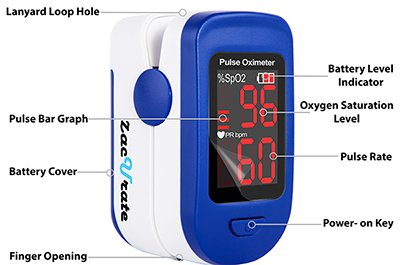Figure 1: The patient places a finger inside this portable pulse oximeter to get a clear indication of pulse oxygen level and heart rate. (Image source: Zacurate)

The blood’s oxygen saturation is calculated using the ratio of oxygenated hemoglobin (HbO2) to the reduced hemoglobin (Hb). To measure the pulse rate, the system collects several samples of the pulsing blood’s waveform. To accurately measure these two parameters, the LEDs’ signal must have both low noise and low distortion.

This article discusses the electronic blocks of a typical pulse oximeter before introducing appropriate LED drive solutions and their application toward the design of a low-noise, low-distortion LED drive circuit.

## Pulse oximeter electronics

The main electronic blocks of a pulse oximeter are the LED transmission circuitry and the photo detecting system. A typical pulse oximeter’s electronics configuration has a pair of LEDs affixed on the top of the finger glove and photodetectors on the bottom (Figure 2).Figure 2: In a pulse oximeter, accurate measurements require low noise and low distortion signals from the red (HbO2) and IR (Hb) drive electronics. (Image source: Bonnie Baker)

In Figure 2, the elements in the two LED driver circuits are a low noise digital-to-analog converter (DAC) with a following LED amplifier driver circuit. The red and IR (infrared) LEDs send alternately pulsed signals from a high current level to a low current level, creating two pulse-width signals that travel through the finger. The timing of the driving signals to these two LEDs are offset so that the receiving photodetector can separate one signal from the other. The time that these current pulses are active high is typically on the order of several hundred microseconds.

The peak wavelengths of the red and IR LEDs are 660 nm (HbO2) and 940 nm (Hb), respectively. Different wavelengths are used because HbO2 and Hb have different spectral responses. A ratio calculation with these two values provides an estimate of the percentage of blood oxygen content (SpO2).

The elements of the transimpedance or I-V photodiode circuit are an operation amplifier, an analog notch filter, and a gain amplifier. Following the gain amplifier is an analog-to-digital converter (ADC) which provides a digital output to the DSP chip.

## LED driver circuit

The pulse oximeter circuit’s signal path starts with the LED driver. The single supply LED driver chain has a voltage reference (U1), a DAC (U3), a DAC output buffer (U4), and a transistor current source (Q1) (Figure 3).Figure 3: A simplified LED driver for a pulse oximeter system with the photodiode receiver (on right). (Image source: Bonnie Baker, using modified Analog Devices material)

The voltage reference (U1) is for the 16 bit DAC and defines the analog output voltage value, per Equation 1.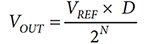Where D = DAC register, the decimal data word, and N = number of DAC bits.

For example, if U1 is Analog Devices’ ADR4525BRZ-R7 series 2.5 volt voltage reference, and U3 is Analog Devices’ AD5542AACPZ-REEL7 16-bit serial DAC, Equation 1 becomes:The resulting DAC mid-scale voltage is 1.25 volts, and the least significant bit (LSB) size is VREF /(2N) = 2.5/65,536 = 38.1 microvolts (µV).

The ADR4525 is designed to be a high precision, low noise (1.25 millivolt (mV) p-p, 0.1 Hz to 10 Hz), temperature stable reference. The device's low output voltage temperature coefficient (2 ppm/°C maximum) and low long-term output voltage drift (25 ppm over 1000 hours at 60˚C) ensures system accuracy over time and temperature variations. The initial room temperature error of the ADR4525B is ±0.02%, maximum.

## LED driver noise analysis

In the LED driver circuit noise analysis, the 16-bit DAC guides the selection of the surrounding devices. In other words, if the DAC resolution is 12 bits, the LSB size is 601.35 mV, which relaxes the noise requirements of the voltage references and operational amplifiers.

In the LED driver circuit, however, the near DC noise and nonlinearity do impact the LED brightness levels. The generation of the near DC noise sources are:

• The DAC’s integral and differential nonlinearity
• The voltage reference’s 1/f noise and the amplifier’s 1/f noise
• The amplifier’s common-mode distortion

These noise sources are worth a closer look.

DAC’s integral and differential nonlinearity: Differential nonlinearity (DNL) is the difference between the actual step size and the ideal value of 1 LSB. A DNL error less than –1 LSB can cause missing codes. The AD5542A 16-bit DAC has a differential error that is within approximately ±0.4 LSB (Figure 4).Figure 4: The AD5542A 16-bit DAC’s differential nonlinearity vs. code shows a differential error that is within approximately ±0.4 LSB. (Image source: Analog Devices)

The integral nonlinearity (INL) error is the maximum deviation of an output voltage from the corresponding output voltage of the ideal transfer curve, with the measured offset and gain errors zeroed. The AD5542A has an INL error that is within approximately -0.6 LSB to 0.25 LSB (Figure 5).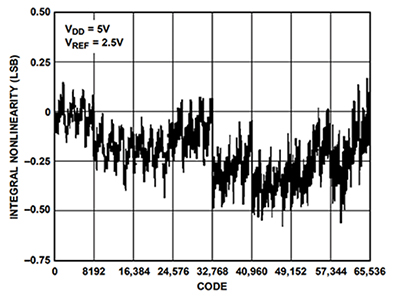Figure 5: A graph of the AD5542A’s integral nonlinearity vs. code shows the integral nonlinearity error is within approximately -0.6 to +0.25 LSB. (Image source: Analog Devices)

From the Figure 4 and Figure 5 nonlinearity graphs, the maximum analog noise can be one-third of the worst-case nonlinearity, or 0.6 LSB which equals: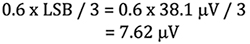1/f voltage reference and amplifier noise: The frequency range of the 1/f noise is equal to 0.1 Hz to 10 Hz. The voltage reference (U1) and the reference buffer (U2) feed directly into the DAC (U3). It is appropriate to use a root-sum-square (RSS) calculation when combining the 1/f noise contribution of U1 and U2 (Equation 3).If U1 is the ADR4525 series 2.5 volt voltage reference, the 1/f noise is 1.25 mVP-P. Additionally, U2 and U4 are each half of Analog Devices’ ADA4500-2, 10 MHz, 14.5 nV/√Hz, rail-to-rail I/O, zero input crossover distortion operational amplifiers. For the ADA4500-2, the 1/f noise is 2 mVP-P.

Using Equation 3, the total 1/f noise going into the DAC REFF pin is: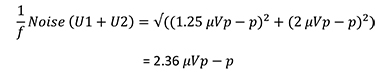The noise for the voltage reference (U1) and the buffer amplifier (U2) is markedly smaller than the DAC’s LSB size.

The amplifier’s common-mode distortion: The input and output swing of the U4 DAC buffer op amp travels from rail-to-rail. A typical rail-to-rail input amplifier achieves rail-to-rail input swing using two differential pairs. At the low common-mode range the bottom differential pair is active, and at the high end the other pair is active. Each differential pair has its own offset voltage. This typical complementary dual differential pair introduces crossover distortion (Figure 6). Similarly, the amplifier’s change in offset voltage causes nonlinearity as a DAC buffer (Figure 7).Figure 6: With two input differential pairs, the offset voltage creates distortion across the common-mode input voltage range. (Image source: Bonnie Baker)Figure 7: DAC nonlinearity with an amplifier output buffer that has two differential input structures. (Image source: Analog Devices)

Figure 7 shows the op amp’s nonlinearity. When the common-mode voltage increases, the active differential pair changes from the P-type pair to the N-type pair causing a crossover distortion. The crossover distortion causes an error swing from +4 LSB to −15 LSB.

However, the ADA4500-2 is not a typical amplifier in that it uses only one differential input pair to achieve the rail-to-rail input swing, so there’s no crossover distortion. It accomplishes this by using a positive voltage charge pump in the input structure to achieve the full rail-to-rail input swing.

The added advantage in this case is that the DAC buffer amplifier (U4) can be formed using the second half of the dual op amp ADA4500-2. As discussed earlier,  the first half is used for U2, the voltage reference’s buffer amplifier.

The output impedance of the DAC (U3) is constant (typically 6.25 kΩ) and code independent. The output buffer (U4) requires a low input bias current and high input impedance to minimize errors. These requirements make the ADA5400-2 a good fit as it has an input bias current of 2 picoamps (pA) at room temperature, a high input impedance, and a maximum input bias current of 190 pA over a temperature range of -40˚C to 125˚C.

## Noise measurements

The target noise for this complete LED driving system is less than 15 mVP-P. The noise contributions, from datasheet specifications, of the selected components are:

• 16-bit DAC
• 0.134 μVP-P
• 2.5 volt output reference
• 1.25 μVP-P
• U2: Amplifier ADA4500-2 (reference buffer):
• Zero common-mode crossover distortion
• 2 μVP-P
• U4: Amplifier ADA4500-2 (DAC buffer): 2 μVP-P
• Zero common-mode crossover distortion
• 2 μVP-P

The datasheet RSS noise of the U1 – U4 components is 3.1 mVP-P.

The actual noise measurement of this circuit is done using a noise measuring box with a gain of 10,000 V/V combined with a 0.1 Hz to 10 Hz filter (Figure 8).Figure 8: Test setup for measuring 0.1 Hz to 10 Hz noise with a gain of 10,000. (Image source: Analog Devices)

The EVAL-CN0370_PMDZ eval kit from Analog Devices generates the measurement data for the circuit (originally shown in Figure 3). With the differential input shorted, the noise output of the box and the noise with the circuit connected is 7.81 mVP-P (Figure 9) and 9.6 mVP-P (Figure 10), respectively.Figure 9: Output noise with input-to-noise measuring box shorted measures 78.1 mVP-P (or 7.81 μVP-P, referenced to input). (Image source: Analog Devices)Figure 10: Output noise with Analog Devices EVAL-CN0370-PMDZ connected measures 96 mVP-P (or 9.6 μVP-P referenced to input). (Image source: Analog Devices)

The uncorrelated noise from the two systems combines with the RSS formula to give: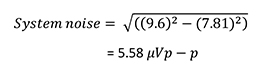The noise current driving the LED is equal to 5.58 mVP-P divided by 124 Ω or 45 nAP-P.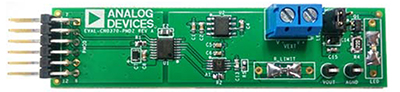Figure 11: The EVAL-CN0370-PMDZ circuit evaluation board is a complete single supply, low noise LED current source driver that is controlled by a 16-bit DAC, all in a PMOD form factor. (Image source: Analog Devices)

## Conclusion

IR and red LEDs are used in pulse oximeters for the noninvasive measurement of blood oxygen saturation and pulse rate. Their role is to illuminate the patient’s finger sufficiently to measure the ratio of HbO2 to Hb, based on the energy received at the photodiode.

The challenge for the system designer is to ensure that the LED is driven by a current with low noise and low distortion. As shown, this challenge is met with the combination of a 16-bit DAC, low noise devices in the 1/f regions, and a rail-to-rail LED driver amplifier with zero crossover distortion.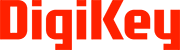Disclaimer: The opinions, beliefs, and viewpoints expressed by the various authors and/or forum participants on this website do not necessarily reflect the opinions, beliefs, and viewpoints of Digi-Key Electronics or official policies of Digi-Key Electronics.